# Other Cohen's Class Time Frequency Distributions (Advanced Signal Processing Toolkit)

LabVIEW 2014 Advanced Signal Processing Toolkit Help

Edition Date: June 2014

Part Number: 372656C-01

»View Product InfoDownload Help (Windows Only)

The short-time Fourier transform (STFT) spectrogram and the Wigner-Ville Distribution (WVD) belong to Cohen's class of time-frequency distributions. In addition to these two prominent Cohen's class methods, the LabVIEW Time Frequency Analysis Tools also provide two other Cohen's class methods—the Choi-Williams Distribution (CWD) and the Cone-Shaped Distribution (CSD).

To understand the other Cohen's class methods, you can start with the ambiguity function, which is equivalent to the 2D inverse Fourier transform of the WVD.

The following figure shows the ambiguity function of the example frequency hopper signal.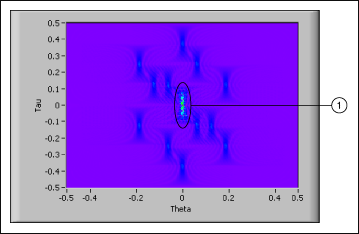1 Representation of Auto-terms

In the previous figure, the components located at the origin of the ambiguity function plane are associated with the auto-terms of the WVD. All the auto-terms overlap at the origin of the ambiguity function plane. The components located away from the origin of the ambiguity function plane correspond to the cross-terms of the WVD. Because the auto-terms and the cross-terms are separated in the ambiguity function plane, you can apply a mask function, also called a kernel function, to keep the auto-terms and remove the cross-terms. The kernel function determines how to suppress the cross-terms. By selecting the appropriate kernel function, you can reduce the cross-term interference and keep some useful properties of the WVD, such as accurate marginal time integration, marginal frequency integration, mean instantaneous frequency, and group delay. Lastly, you can apply the 2D Fourier transform to the ambiguity function to obtain the smooth WVD, also called the Cohen's class time-frequency representation.

The size of the ambiguity function quadratically increases with the length of the input signal. Therefore, large signals require a long computation time and more memory. If you need to analyze large signals, divide the signal into smaller segments and analyze each segment individually.

## Choi-Williams Distribution

The following CWD uses an exponential kernel function.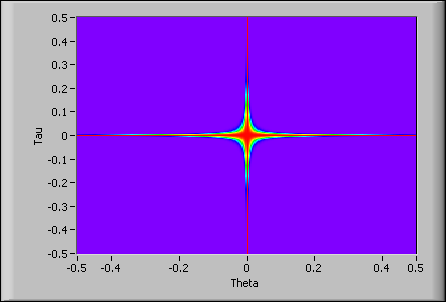The exponential kernel function has the same dimensions as the ambiguity function. The exponential kernel function suppresses the cross-terms away from the horizontal axis and the vertical axis. Therefore, the CWD reduces the cross-terms generated by two auto-terms with different time centers and frequency centers. The exponential kernel function does not reduce the values of the ambiguity function on the horizontal axis or the vertical axis. Therefore, the CWD still possesses the useful properties of the WVD, such as accurate marginal time integration, marginal frequency integration, mean instantaneous frequency, and group delay.

The CWD has a coarser time-frequency resolution than the WVD because the CWD also blurs the auto-terms when the CWD reduces the cross-terms. Because the exponential kernel function does not reduce the values of the ambiguity function on the horizontal axis or the vertical axis, the CWD preserves the cross-terms on the horizontal axis and the vertical axis. In other words, the CWD does not suppress the cross-terms that two auto-terms with the same time center or frequency center generate.

Use the TFA Choi-Williams Distribution VI to compute the CWD.

The following figure shows the CWD of the example frequency hopper signal.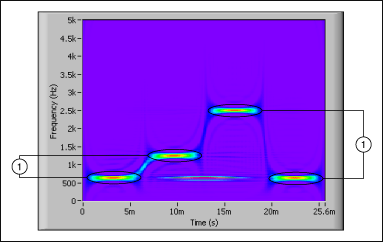1 Auto-terms

The exponential kernel function includes an alpha parameter to balance the cross-term suppression and the blurriness of auto-terms. The larger the value of the alpha parameter, the better the cross-term suppression and the more blurry auto-terms become. As alpha approaches zero, the CWD converges to the WVD.

## Cone-Shaped Distribution

The following CSD uses the cone-shaped kernel function.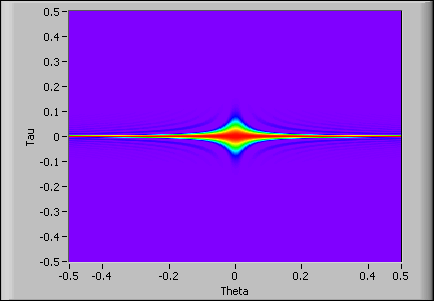The cone-shaped kernel function suppresses the cross-terms away from the vertical axis and the origin of the ambiguity function plane. Therefore, the CSD suppresses the cross-terms that two auto-terms with different time centers and frequency centers generate. Additionally, the CSD suppresses the cross-terms that two auto-terms with the same frequency center generate.

Use the TFA Cone-Shaped Distribution VI to compute the CSD.

The following figure shows the CSD of the example frequency hopper signal with all cross-terms suppressed.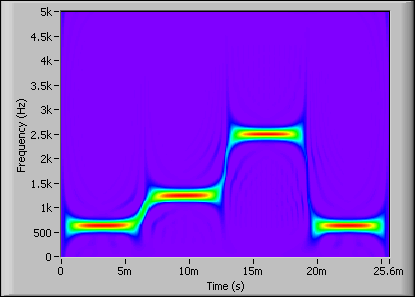The cone-shaped kernel function does not suppress the values of the ambiguity function on the horizontal axis. Therefore, the CSD cannot reduce cross-terms that two auto-terms with the same time center generate. The CSD provides accurate marginal time integration and mean instantaneous frequency but does not provide accurate marginal frequency integration or group delay because the cone-shaped kernel function is not constant on the frequency shift axis.

Similar to the exponential kernel function for the CWD, the cone-shaped kernel function includes an alpha parameter to balance the cross-term suppression and the blurriness of auto-terms. The larger the value of the alpha parameter, the better the cross-term suppression and the more blurry auto-terms become.